# RD Sharma Solutions for Class 11 Chapter 3 - Functions Exercise 3.4

In this section, we shall discuss some standard real functions which frequently occur in the study of calculus and we shall introduce various operations, namely addition, subtraction, multiplication, division etc. on real functions. RD Sharma Class 11 Solutions are prepared in a comprehensive manner to make it interesting for students to solve. Students are advised to practice the solutions on a daily basis which helps them in remembering the concepts for a long time. Exercise solutions of this chapter are provided in the pdf format, which can be downloaded from the links provided below.

## Download the Pdf of RD Sharma Solutions for Class 11 Maths Exercise 3.4 Chapter 3 – Functions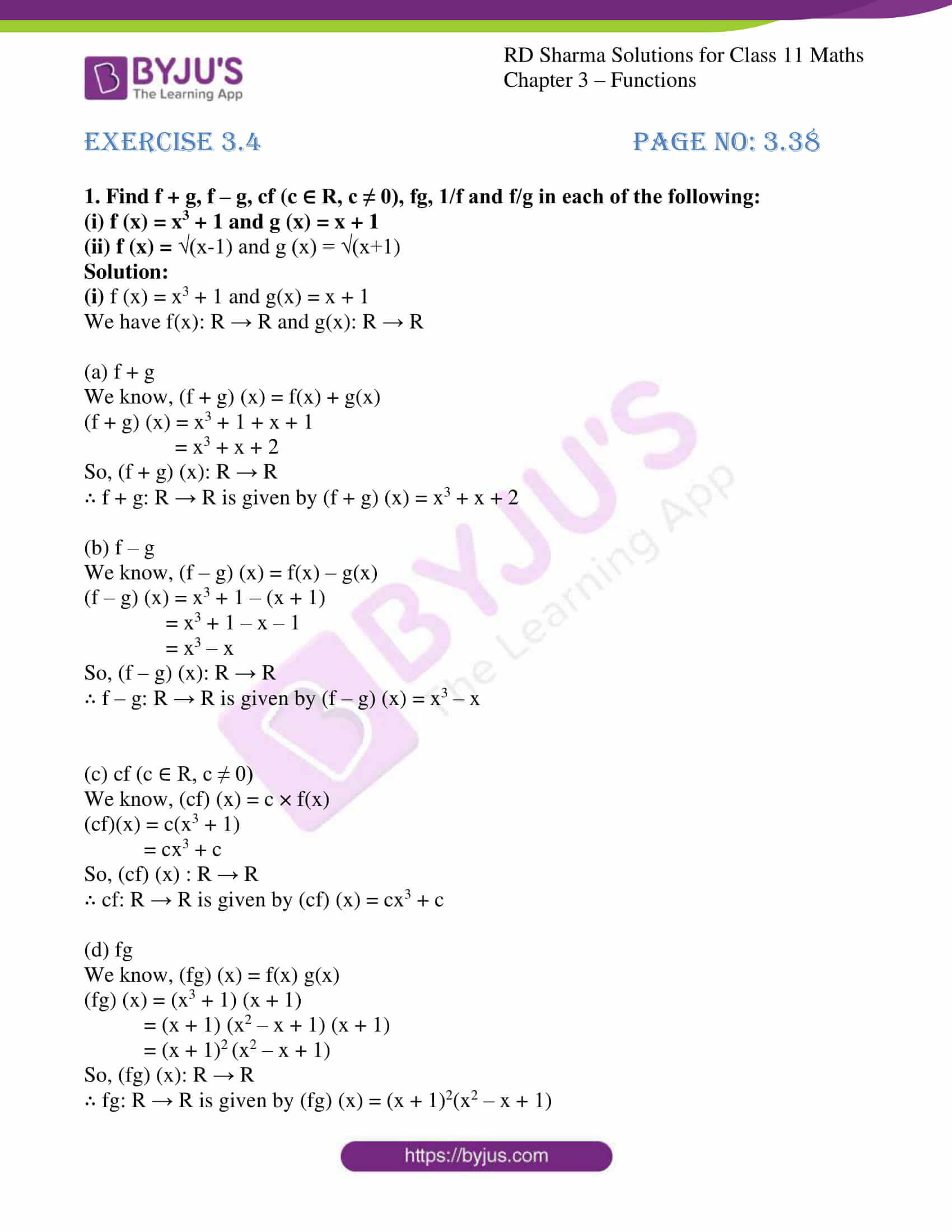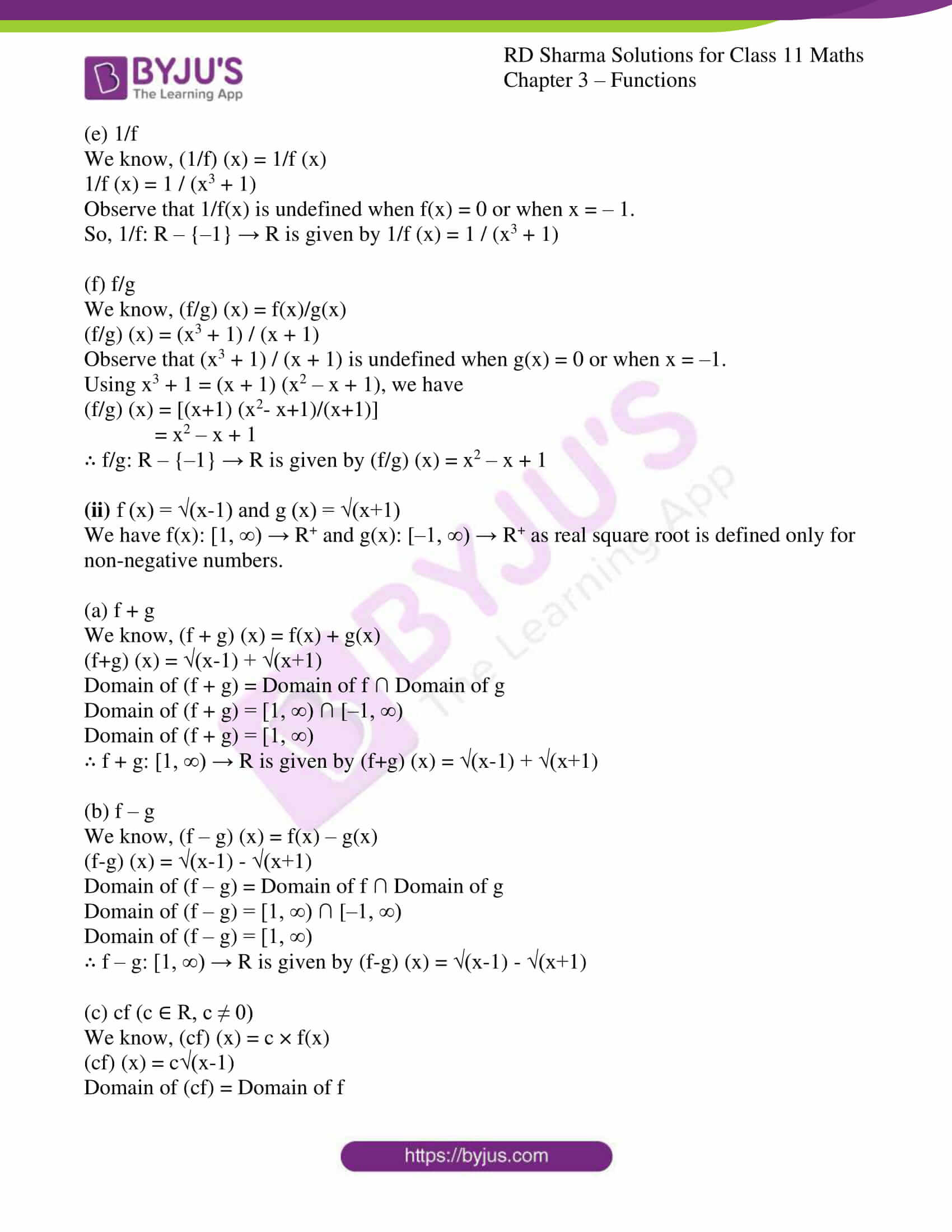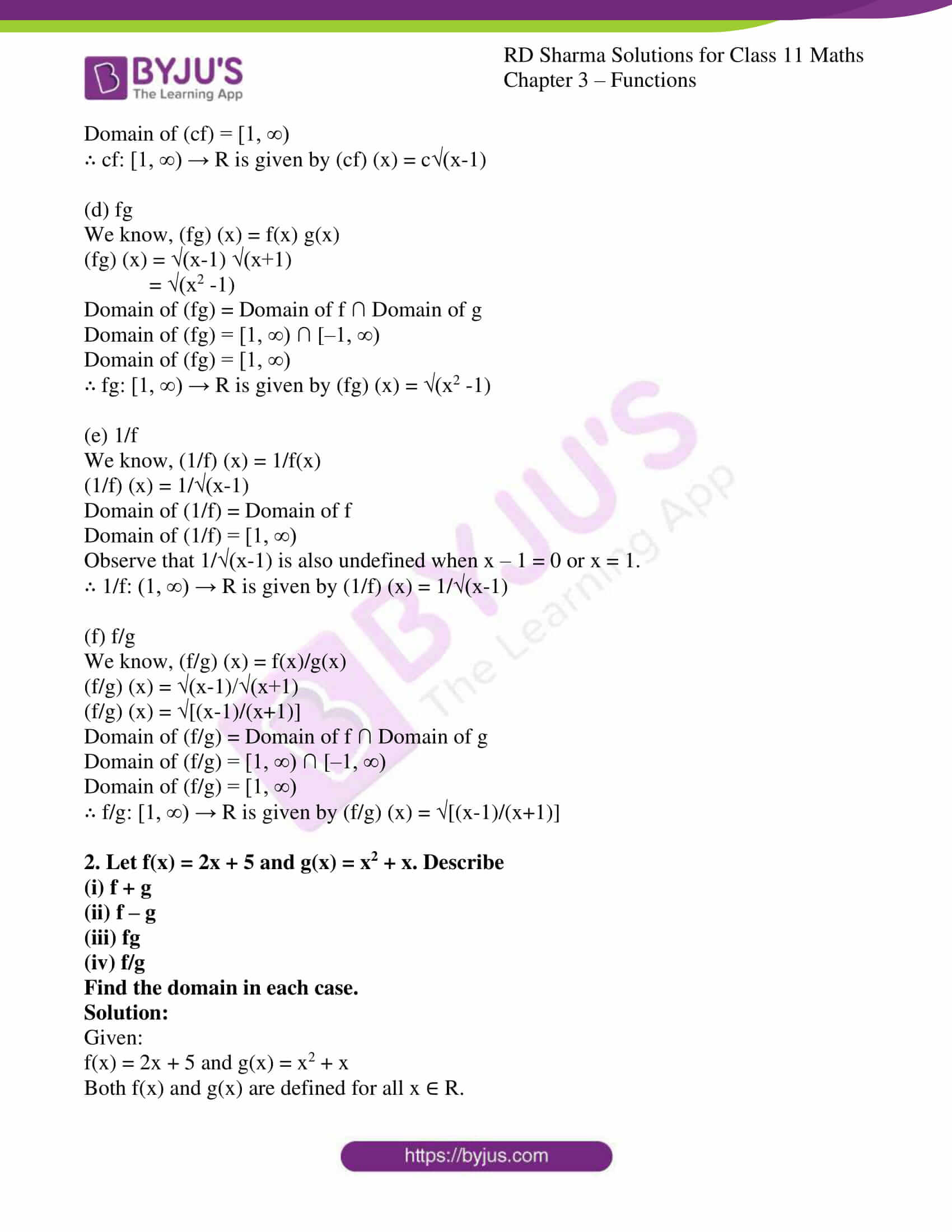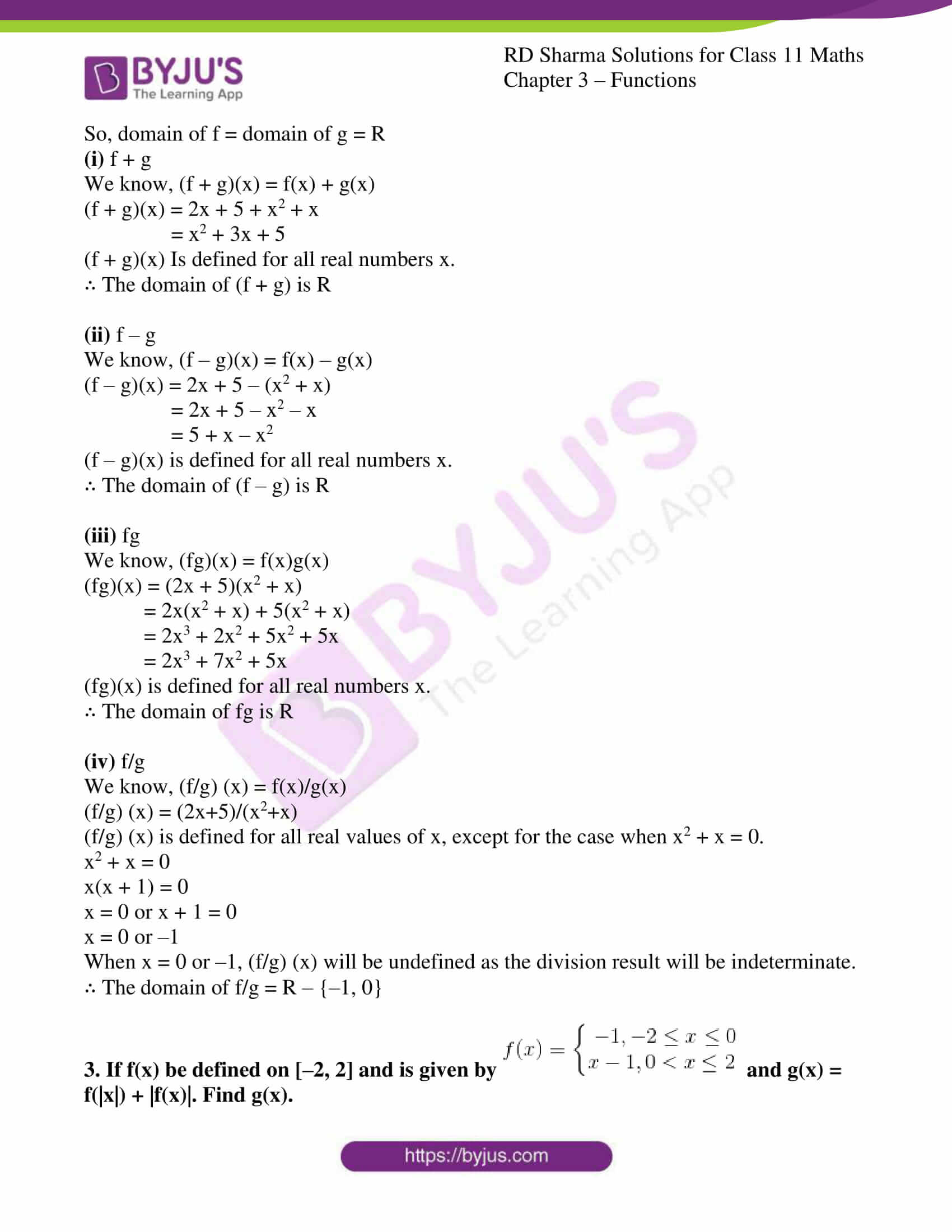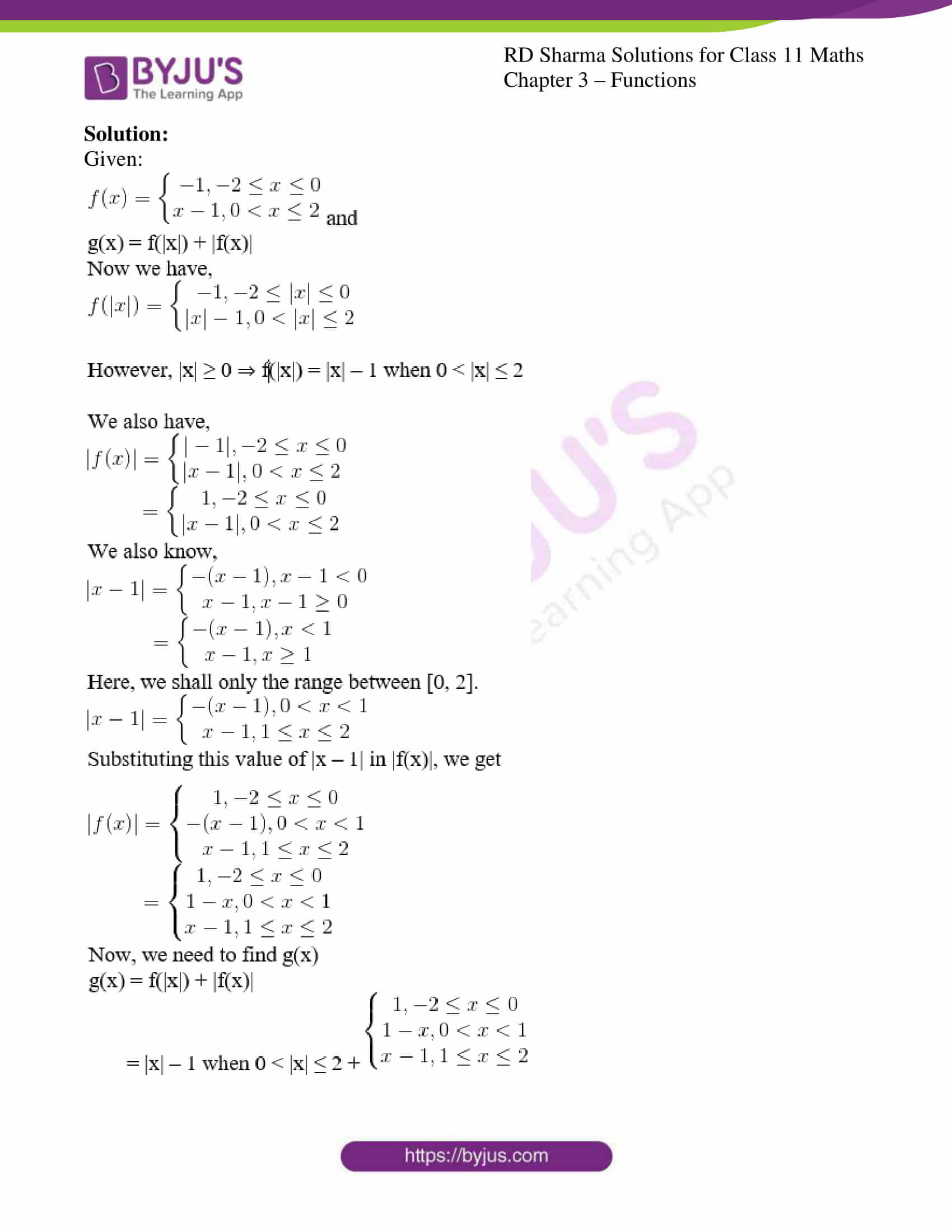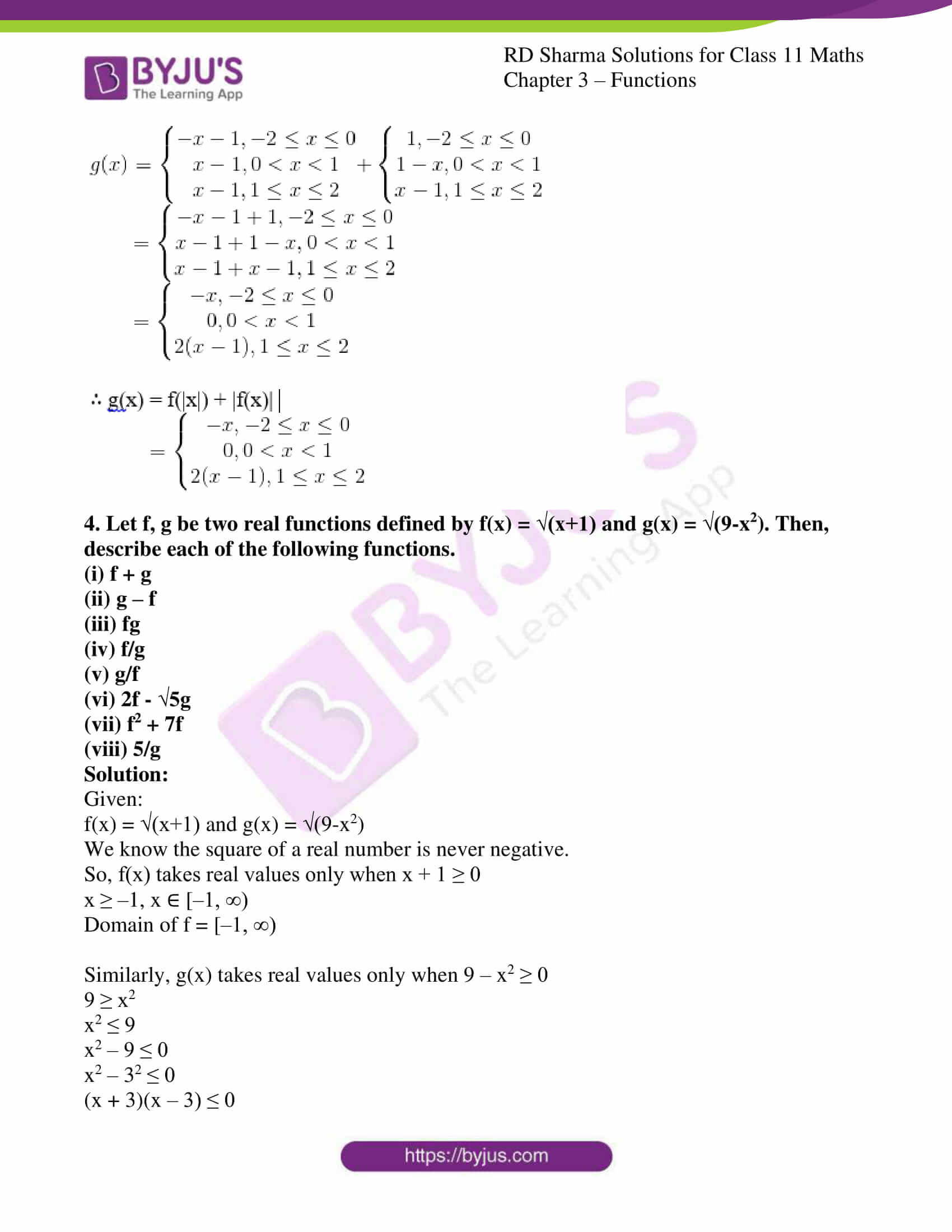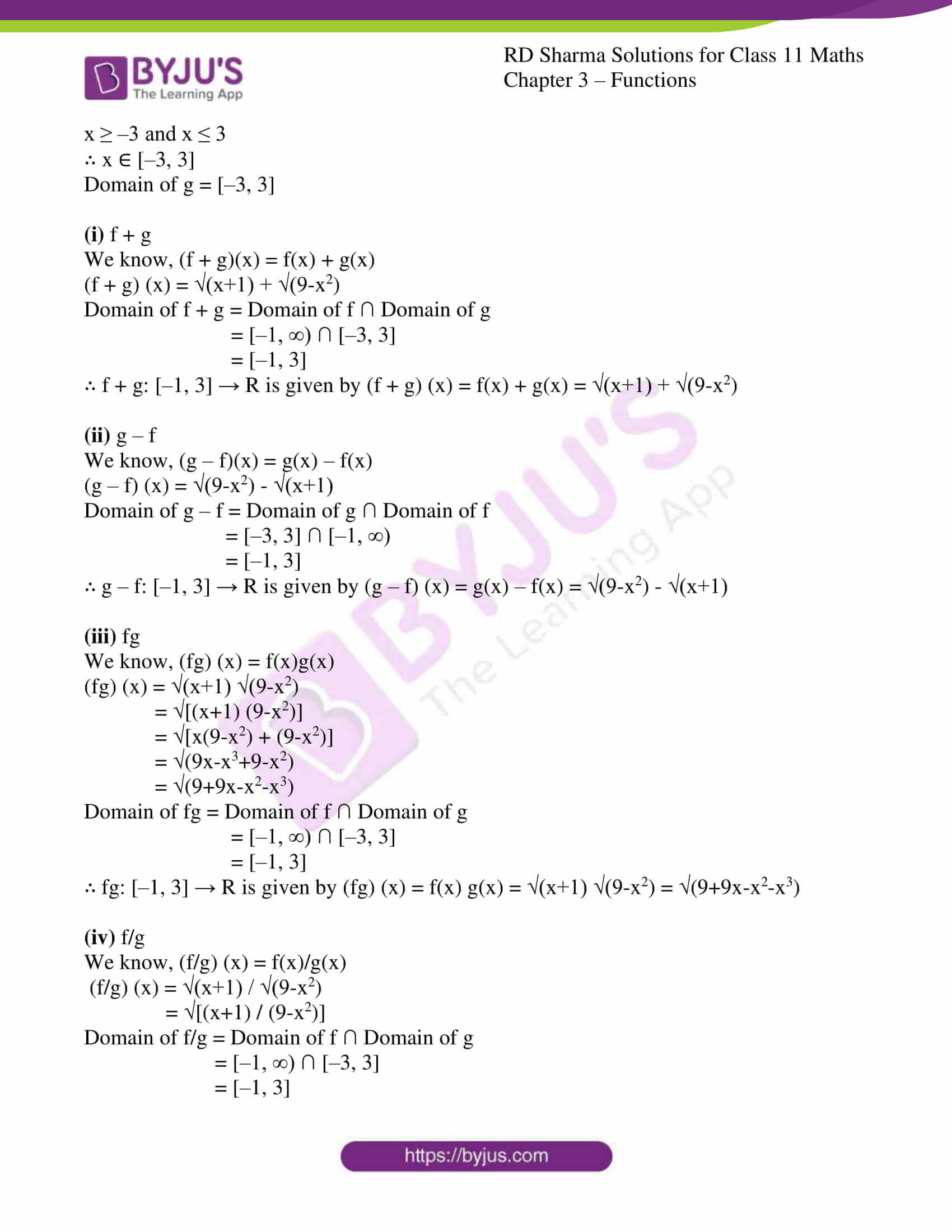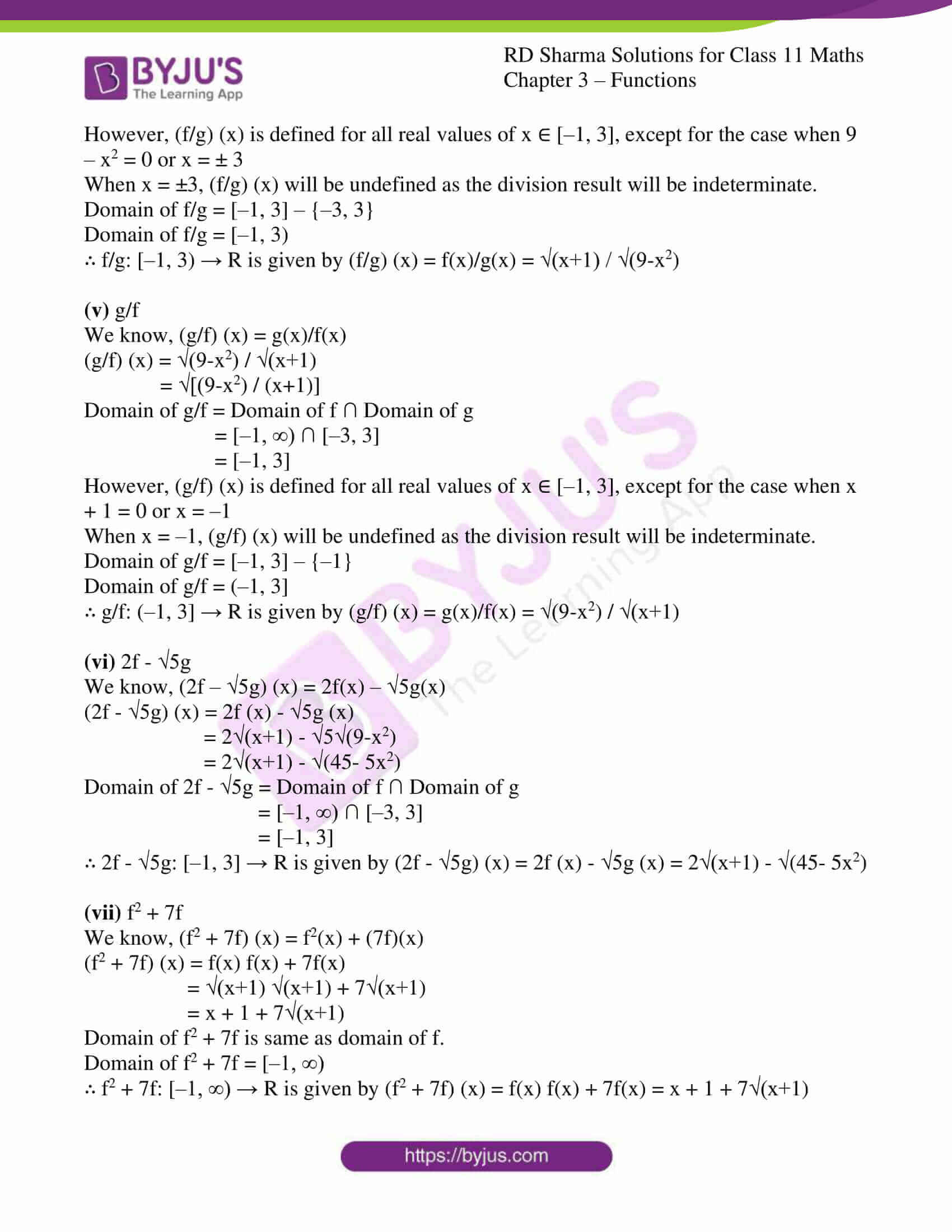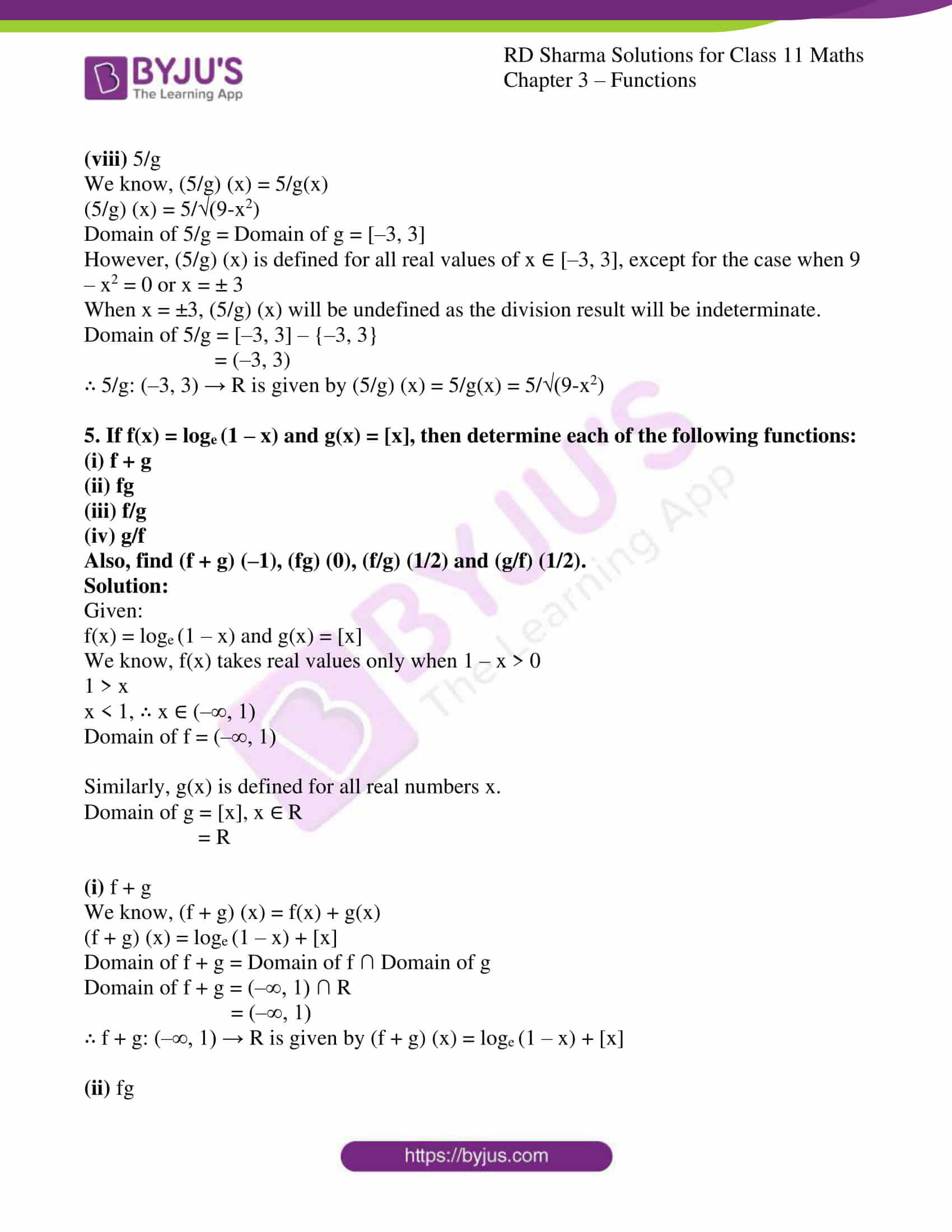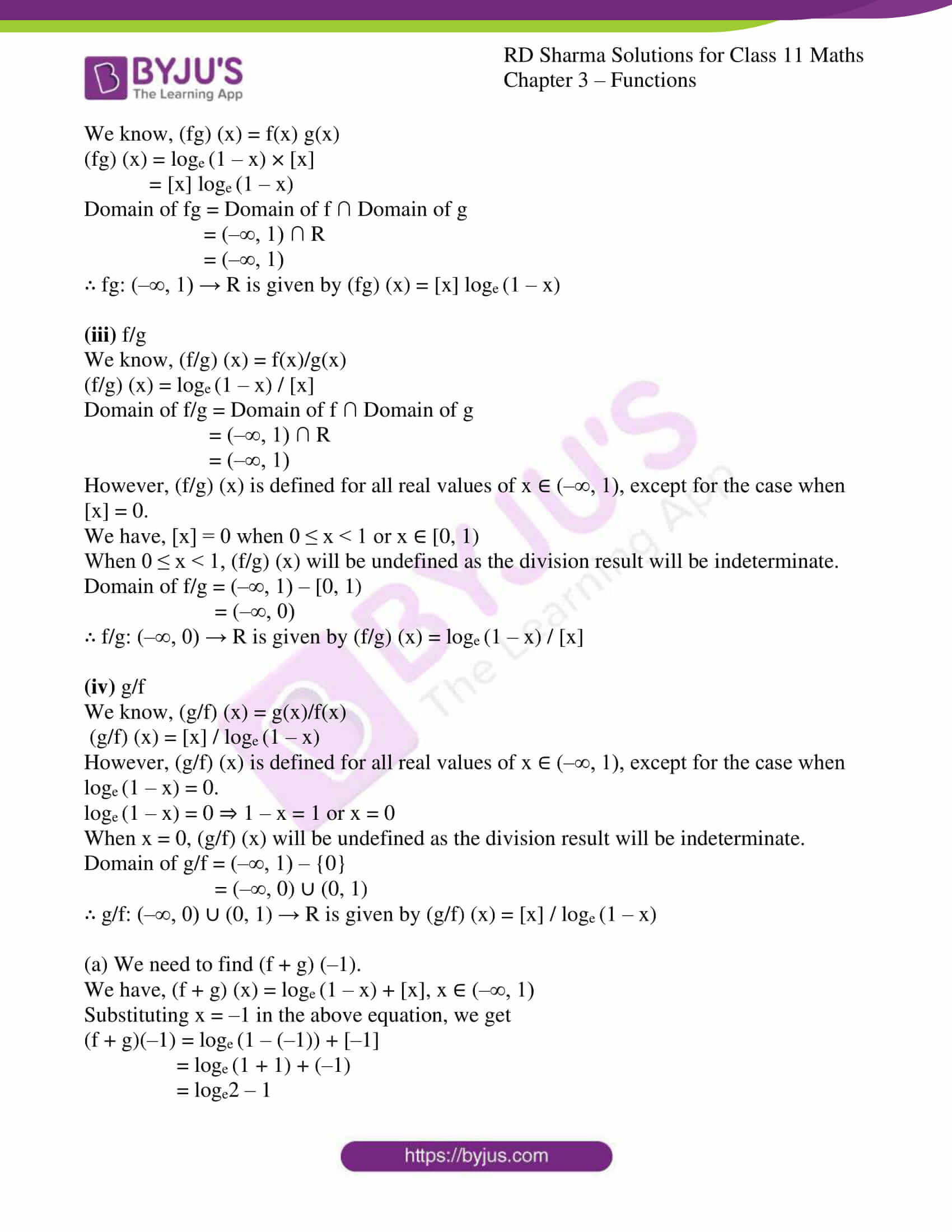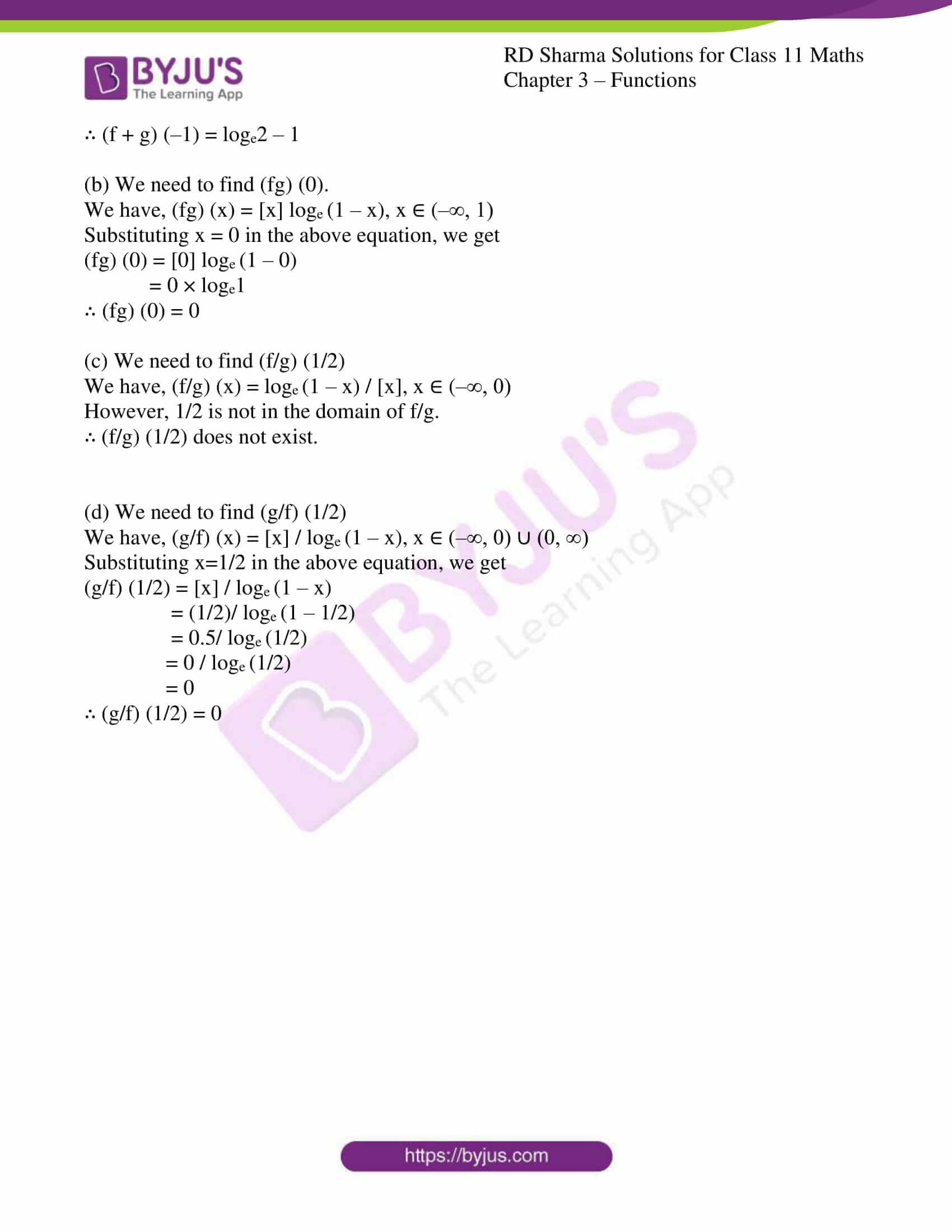### Access answers to RD Sharma Solutions for Class 11 Maths Exercise 3.4 Chapter 3 – Functions

1. Find f + g, f – g, cf (c ∈ R, c ≠ 0), fg, 1/f and f/g in each of the following:
(i) f (x) = x3 + 1 and g (x) = x + 1

(ii) f (x) = √(x-1) and g (x) = √(x+1)

Solution:

(i) f (x) = x3 + 1 and g(x) = x + 1

We have f(x): R → R and g(x): R → R

(a) f + g

We know, (f + g) (x) = f(x) + g(x)

(f + g) (x) = x3 + 1 + x + 1

= x3 + x + 2

So, (f + g) (x): R → R

∴ f + g: R → R is given by (f + g) (x) = x3 + x + 2

(b) f – g

We know, (f – g) (x) = f(x) – g(x)

(f – g) (x) = x3 + 1 – (x + 1)

= x3 + 1 – x – 1

= x3 – x

So, (f – g) (x): R → R

∴ f – g: R → R is given by (f – g) (x) = x3 – x

(c) cf (c ∈ R, c ≠ 0)

We know, (cf) (x) = c × f(x)

(cf)(x) = c(x3 + 1)

= cx3 + c

So, (cf) (x) : R → R

∴ cf: R → R is given by (cf) (x) = cx3 + c

(d) fg

We know, (fg) (x) = f(x) g(x)

(fg) (x) = (x3 + 1) (x + 1)

= (x + 1) (x2 – x + 1) (x + 1)

= (x + 1)2 (x2 – x + 1)

So, (fg) (x): R → R

∴ fg: R → R is given by (fg) (x) = (x + 1)2(x2 – x + 1)

(e) 1/f

We know, (1/f) (x) = 1/f (x)

1/f (x) = 1 / (x3 + 1)

Observe that 1/f(x) is undefined when f(x) = 0 or when x = – 1.

So, 1/f: R – {–1} → R is given by 1/f (x) = 1 / (x3 + 1)

(f) f/g

We know, (f/g) (x) = f(x)/g(x)

(f/g) (x) = (x3 + 1) / (x + 1)

Observe that (x3 + 1) / (x + 1) is undefined when g(x) = 0 or when x = –1.

Using x3 + 1 = (x + 1) (x2 – x + 1), we have

(f/g) (x) = [(x+1) (x2– x+1)/(x+1)]

= x2 – x + 1

∴ f/g: R – {–1} → R is given by (f/g) (x) = x2 – x + 1

(ii) f (x) = √(x-1) and g (x) = √(x+1)

We have f(x): [1, ∞) → R+ and g(x): [–1, ∞) → R+ as real square root is defined only for non-negative numbers.

(a) f + g

We know, (f + g) (x) = f(x) + g(x)

(f+g) (x) = √(x-1) + √(x+1)

Domain of (f + g) = Domain of f ∩ Domain of g

Domain of (f + g) = [1, ∞) ∩ [–1, ∞)

Domain of (f + g) = [1, ∞)

∴ f + g: [1, ∞) → R is given by (f+g) (x) = √(x-1) + √(x+1)

(b) f – g

We know, (f – g) (x) = f(x) – g(x)

(f-g) (x) = √(x-1) – √(x+1)

Domain of (f – g) = Domain of f ∩ Domain of g

Domain of (f – g) = [1, ∞) ∩ [–1, ∞)

Domain of (f – g) = [1, ∞)

∴ f – g: [1, ∞) → R is given by (f-g) (x) = √(x-1) – √(x+1)

(c) cf (c ∈ R, c ≠ 0)

We know, (cf) (x) = c × f(x)

(cf) (x) = c√(x-1)

Domain of (cf) = Domain of f

Domain of (cf) = [1, ∞)

∴ cf: [1, ∞) → R is given by (cf) (x) = c√(x-1)

(d) fg

We know, (fg) (x) = f(x) g(x)

(fg) (x) = √(x-1) √(x+1)

= √(x2 -1)

Domain of (fg) = Domain of f ∩ Domain of g

Domain of (fg) = [1, ∞) ∩ [–1, ∞)

Domain of (fg) = [1, ∞)

∴ fg: [1, ∞) → R is given by (fg) (x) = √(x2 -1)

(e) 1/f

We know, (1/f) (x) = 1/f(x)

(1/f) (x) = 1/√(x-1)

Domain of (1/f) = Domain of f

Domain of (1/f) = [1, ∞)

Observe that 1/√(x-1) is also undefined when x – 1 = 0 or x = 1.

∴ 1/f: (1, ∞) → R is given by (1/f) (x) = 1/√(x-1)

(f) f/g

We know, (f/g) (x) = f(x)/g(x)

(f/g) (x) = √(x-1)/√(x+1)

(f/g) (x) = √[(x-1)/(x+1)]

Domain of (f/g) = Domain of f ∩ Domain of g

Domain of (f/g) = [1, ∞) ∩ [–1, ∞)

Domain of (f/g) = [1, ∞)

∴ f/g: [1, ∞) → R is given by (f/g) (x) = √[(x-1)/(x+1)]

2. Let f(x) = 2x + 5 and g(x) = x2 + x. Describe

(i) f + g
(ii) f – g
(iii) fg
(iv) f/g
Find the domain in each case.

Solution:

Given:

f(x) = 2x + 5 and g(x) = x2 + x

Both f(x) and g(x) are defined for all x ∈ R.

So, domain of f = domain of g = R

(i) f + g

We know, (f + g)(x) = f(x) + g(x)

(f + g)(x) = 2x + 5 + x2 + x

= x2 + 3x + 5

(f + g)(x) Is defined for all real numbers x.

∴ The domain of (f + g) is R

(ii) f – g

We know, (f – g)(x) = f(x) – g(x)

(f – g)(x) = 2x + 5 – (x2 + x)

= 2x + 5 – x2 – x

= 5 + x – x2

(f – g)(x) is defined for all real numbers x.

∴ The domain of (f – g) is R

(iii) fg

We know, (fg)(x) = f(x)g(x)

(fg)(x) = (2x + 5)(x2 + x)

= 2x(x2 + x) + 5(x2 + x)

= 2x3 + 2x2 + 5x2 + 5x

= 2x3 + 7x2 + 5x

(fg)(x) is defined for all real numbers x.

∴ The domain of fg is R

(iv) f/g

We know, (f/g) (x) = f(x)/g(x)

(f/g) (x) = (2x+5)/(x2+x)

(f/g) (x) is defined for all real values of x, except for the case when x2 + x = 0.

x2 + x = 0

x(x + 1) = 0

x = 0 or x + 1 = 0

x = 0 or –1

When x = 0 or –1, (f/g) (x) will be undefined as the division result will be indeterminate.

∴ The domain of f/g = R – {–1, 0}

3. If f(x) be defined on [–2, 2] and is given by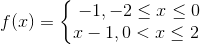and g(x) = f(|x|) + |f(x)|. Find g(x).

Solution:

Given: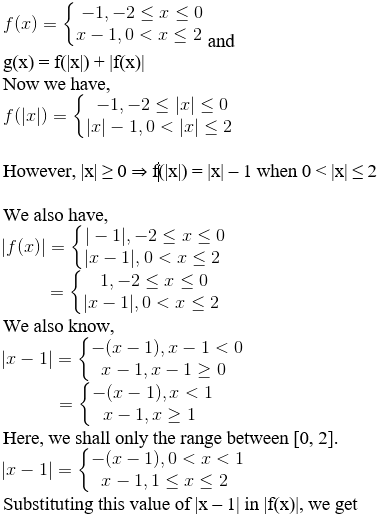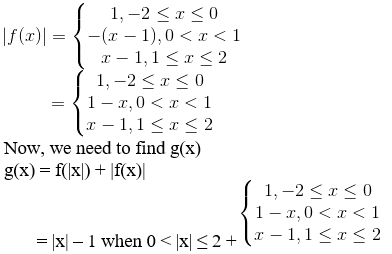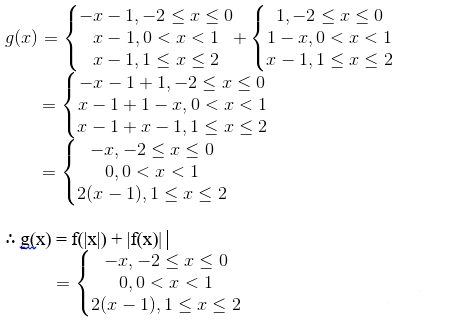4. Let f, g be two real functions defined by f(x) = √(x+1) and g(x) = √(9-x2). Then, describe each of the following functions.
(i) f + g
(ii) g – f
(iii) fg
(iv) f/g
(v) g/f
(vi) 2f – √5g
(vii) f2 + 7f
(viii) 5/g

Solution:

Given:

f(x) = √(x+1) and g(x) = √(9-x2)

We know the square of a real number is never negative.

So, f(x) takes real values only when x + 1 ≥ 0

x ≥ –1, x ∈ [–1, ∞)

Domain of f = [–1, ∞)

Similarly, g(x) takes real values only when 9 – x2 ≥ 0

9 ≥ x2

x2 ≤ 9

x2 – 9 ≤ 0

x2 – 32 ≤ 0

(x + 3)(x – 3) ≤ 0

x ≥ –3 and x ≤ 3

∴ x ∈ [–3, 3]

Domain of g = [–3, 3]

(i) f + g

We know, (f + g)(x) = f(x) + g(x)

(f + g) (x) = √(x+1) + √(9-x2)

Domain of f + g = Domain of f ∩ Domain of g

= [–1, ∞) ∩ [–3, 3]

= [–1, 3]

∴ f + g: [–1, 3] → R is given by (f + g) (x) = f(x) + g(x) = √(x+1) + √(9-x2

(ii) g – f

We know, (g – f)(x) = g(x) – f(x)

(g – f) (x) = √(9-x2) – √(x+1)

Domain of g – f = Domain of g ∩ Domain of f

= [–3, 3] ∩ [–1, ∞)

= [–1, 3]

∴ g – f: [–1, 3] → R is given by (g – f) (x) = g(x) – f(x) = √(9-x2) – √(x+1)

(iii) fg

We know, (fg) (x) = f(x)g(x)

(fg) (x) = √(x+1) √(9-x2)

= √[(x+1) (9-x2)]

= √[x(9-x2) + (9-x2)]

= √(9x-x3+9-x2)

= √(9+9x-x2-x3)

Domain of fg = Domain of f ∩ Domain of g

= [–1, ∞) ∩ [–3, 3]

= [–1, 3]

∴ fg: [–1, 3] → R is given by (fg) (x) = f(x) g(x) = √(x+1) √(9-x2) = √(9+9x-x2-x3)

(iv) f/g

We know, (f/g) (x) = f(x)/g(x)

(f/g) (x) = √(x+1) / √(9-x2)

= √[(x+1) / (9-x2)]

Domain of f/g = Domain of f ∩ Domain of g

= [–1, ∞) ∩ [–3, 3]

= [–1, 3]

However, (f/g) (x) is defined for all real values of x ∈ [–1, 3], except for the case when 9 – x2 = 0 or x = ± 3

When x = ±3, (f/g) (x) will be undefined as the division result will be indeterminate.

Domain of f/g = [–1, 3] – {–3, 3}

Domain of f/g = [–1, 3)

∴ f/g: [–1, 3) → R is given by (f/g) (x) = f(x)/g(x) = √(x+1) / √(9-x2)

(v) g/f

We know, (g/f) (x) = g(x)/f(x)

(g/f) (x) = √(9-x2) / √(x+1)

= √[(9-x2) / (x+1)]

Domain of g/f = Domain of f ∩ Domain of g

= [–1, ∞) ∩ [–3, 3]

= [–1, 3]
However, (g/f) (x) is defined for all real values of x ∈ [–1, 3], except for the case when x + 1 = 0 or x = –1

When x = –1, (g/f) (x) will be undefined as the division result will be indeterminate.

Domain of g/f = [–1, 3] – {–1}

Domain of g/f = (–1, 3]

∴ g/f: (–1, 3] → R is given by (g/f) (x) = g(x)/f(x) = √(9-x2) / √(x+1)

(vi) 2f – √5g

We know, (2f – √5g) (x) = 2f(x) – √5g(x)

(2f – √5g) (x) = 2f (x) – √5g (x)

= 2√(x+1) – √5√(9-x2)

= 2√(x+1) – √(45- 5x2)

Domain of 2f – √5g = Domain of f ∩ Domain of g

= [–1, ∞) ∩ [–3, 3]

= [–1, 3]

∴ 2f – √5g: [–1, 3] → R is given by (2f – √5g) (x) = 2f (x) – √5g (x) = 2√(x+1) – √(45- 5x2)

(vii) f2 + 7f

We know, (f2 + 7f) (x) = f2(x) + (7f)(x)

(f2 + 7f) (x) = f(x) f(x) + 7f(x)

= √(x+1) √(x+1) + 7√(x+1)

= x + 1 + 7√(x+1)

Domain of f2 + 7f is same as domain of f.

Domain of f2 + 7f = [–1, ∞)

∴ f2 + 7f: [–1, ∞) → R is given by (f2 + 7f) (x) = f(x) f(x) + 7f(x) = x + 1 + 7√(x+1)

(viii) 5/g

We know, (5/g) (x) = 5/g(x)

(5/g) (x) = 5/√(9-x2)

Domain of 5/g = Domain of g = [–3, 3]

However, (5/g) (x) is defined for all real values of x ∈ [–3, 3], except for the case when 9 – x2 = 0 or x = ± 3

When x = ±3, (5/g) (x) will be undefined as the division result will be indeterminate.

Domain of 5/g = [–3, 3] – {–3, 3}

= (–3, 3)

∴ 5/g: (–3, 3) → R is given by (5/g) (x) = 5/g(x) = 5/√(9-x2

5. If f(x) = loge (1 – x) and g(x) = [x], then determine each of the following functions:
(i) f + g
(ii) fg

(iii) f/g
(iv) g/f

Also, find (f + g) (–1), (fg) (0), (f/g) (1/2) and (g/f) (1/2).

Solution:

Given:

f(x) = loge (1 – x) and g(x) = [x]

We know, f(x) takes real values only when 1 – x > 0

1 > x

x < 1, ∴ x ∈ (–∞, 1)

Domain of f = (–∞, 1)

Similarly, g(x) is defined for all real numbers x.

Domain of g = [x], x ∈ R

= R

(i) f + g

We know, (f + g) (x) = f(x) + g(x)

(f + g) (x) = loge (1 – x) + [x]

Domain of f + g = Domain of f ∩ Domain of g

Domain of f + g = (–∞, 1) ∩ R

= (–∞, 1)

∴ f + g: (–∞, 1) → R is given by (f + g) (x) = loge (1 – x) + [x]

(ii) fg

We know, (fg) (x) = f(x) g(x)

(fg) (x) = loge (1 – x) × [x]

= [x] loge (1 – x)

Domain of fg = Domain of f ∩ Domain of g

= (–∞, 1) ∩ R

= (–∞, 1)

∴ fg: (–∞, 1) → R is given by (fg) (x) = [x] loge (1 – x)

(iii) f/g

We know, (f/g) (x) = f(x)/g(x)

(f/g) (x) = loge (1 – x) / [x]
Domain of f/g = Domain of f ∩ Domain of g

= (–∞, 1) ∩ R

= (–∞, 1)

However, (f/g) (x) is defined for all real values of x ∈ (–∞, 1), except for the case when [x] = 0.

We have, [x] = 0 when 0 ≤ x < 1 or x ∈ [0, 1)

When 0 ≤ x < 1, (f/g) (x) will be undefined as the division result will be indeterminate.

Domain of f/g = (–∞, 1) – [0, 1)

= (–∞, 0)

∴ f/g: (–∞, 0) → R is given by (f/g) (x) = loge (1 – x) / [x]

(iv) g/f

We know, (g/f) (x) = g(x)/f(x)

(g/f) (x) = [x] / loge (1 – x)

However, (g/f) (x) is defined for all real values of x ∈ (–∞, 1), except for the case when loge (1 – x) = 0.

loge (1 – x) = 0 ⇒ 1 – x = 1 or x = 0

When x = 0, (g/f) (x) will be undefined as the division result will be indeterminate.

Domain of g/f = (–∞, 1) – {0}

= (–∞, 0) ∪ (0, 1)

∴ g/f: (–∞, 0) ∪ (0, 1) → R is given by (g/f) (x) = [x] / loge (1 – x)

(a) We need to find (f + g) (–1).

We have, (f + g) (x) = loge (1 – x) + [x], x ∈ (–∞, 1)

Substituting x = –1 in the above equation, we get

(f + g)(–1) = loge (1 – (–1)) + [–1]

= loge (1 + 1) + (–1)

= loge2 – 1

∴ (f + g) (–1) = loge2 – 1

(b) We need to find (fg) (0).

We have, (fg) (x) = [x] loge (1 – x), x ∈ (–∞, 1)

Substituting x = 0 in the above equation, we get

(fg) (0) =  loge (1 – 0)

= 0 × loge1

∴ (fg) (0) = 0

(c) We need to find (f/g) (1/2)

We have, (f/g) (x) = loge (1 – x) / [x], x ∈ (–∞, 0)

However, 1/2 is not in the domain of f/g.

∴ (f/g) (1/2) does not exist.

(d) We need to find (g/f) (1/2)

We have, (g/f) (x) = [x] / loge (1 – x), x ∈ (–∞, 0) ∪ (0, ∞)

Substituting x=1/2 in the above equation, we get

(g/f) (1/2) = [x] / loge (1 – x)

= (1/2)/ loge (1 – 1/2)

= 0.5/ loge (1/2)

= 0 / loge (1/2)

= 0

∴ (g/f) (1/2) = 0